# Chord Of A Circle, Its Length and Theorems

## Chord of a Circle Definition

The chord of a circle can be defined as the line segment joining any two points on the circumference of the circle. It should be noted that the diameter is the longest chord of a circle which passes through the center of the circle. The figure below depicts a circle and its chord.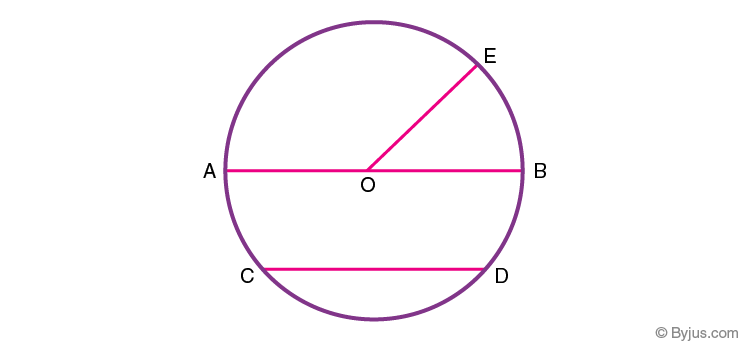In the given circle with ‘O’ as the center, AB represents the diameter of the circle (longest chord), ‘OE’ denotes the radius of the circle and CD represents a chord of the circle.

Let us consider the chord CD of the circle and two points P and Q anywhere on the circumference of the circle except the chord as shown in the figure below. If the endpoints of the chord CD are joined to the point P, then the angle ∠CPD is known as the angle subtended by the chord CD at point P. The angle ∠CQD is the angle subtended by chord CD at Q. The angle ∠COD is the angle subtended by chord CD at the center O.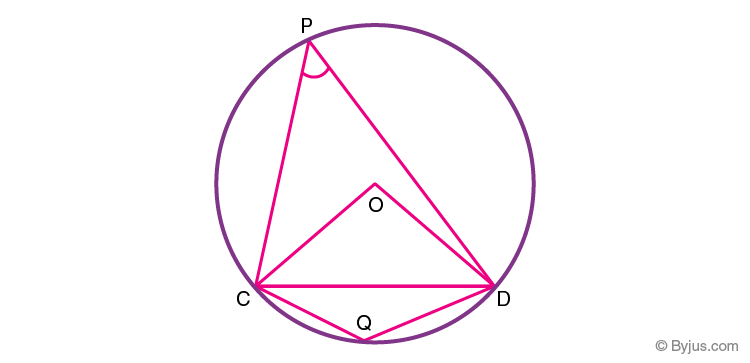## Chord Length Formula

There are two basic formulas to find the length of the chord of a circle which are:

Formula to Calculate Length of a Chord
Chord Length Using Perpendicular Distance from the Center Chord Length = 2 × √(r2 − d2)
Chord Length Using Trigonometry Chord Length = 2 × r × sin(c/2)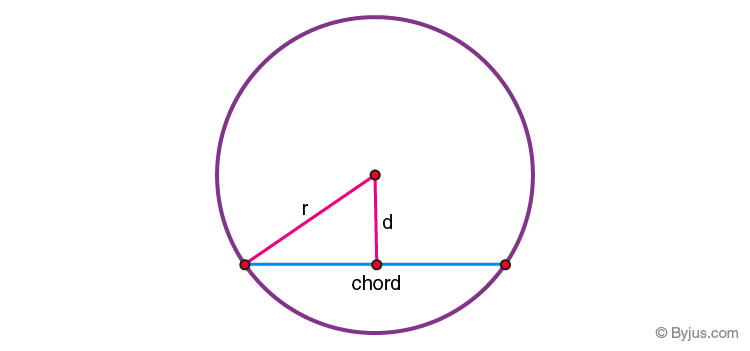Where,

• r is the radius of the circle
• c is the angle subtended at the center by the chord
• d is the perpendicular distance from the chord to the circle center

### Example Question Using Chord Length Formula

Question: Find the length of the chord of a circle where the radius is 7 cm and perpendicular distance from the chord to the center is 4 cm.

Solution:

Given radius, r = 7 cm

and distance, d = 4 cm

Chord length = 2√(r2−d2)

⇒ Chord length = 2√(72−42)

⇒ Chord length = 2√(49−16)

⇒ Chord length = 2√33

⇒ Chord length = 2×5.744

Or , chord length = 11.48 cm

### Video Related to Chords### Chord of a Circle Theorems

If we try to establish a relationship between different chords and the angle subtended by them in the center of the circle, we see that the longer chord subtends a greater angle at the center. Similarly, two chords of equal length subtend equal angle at the center. Let us try to prove this statement.

### Theorem 1: Equal Chords Equal Angles Theorem

Statement: Chords which are equal in length subtend equal angles at the center of the circle.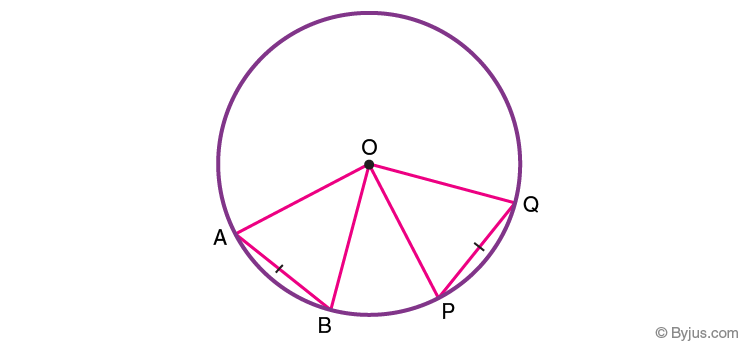Proof:

From fig. 3, In ∆AOB and ∆POQ

S.No. Statement Reason
1. AB=PQ Chords of equal length (Given)
2. OA = OB = OP = OQ Radius of the same circle
3. △AOB ≅ △POQ SSS axiom of Congruence
4. ∠AOB = ∠POQ By CPCT from statement 3

Note: CPCT stands for congruent parts of congruent triangles.

The converse of theorem 1 also holds true, which states that if two angles subtended by two chords at the center are equal then the chords are of equal length. From fig. 3, if ∠AOB =∠POQ, then AB=PQ. Let us try to prove this statement.

### Theorem 2: Equal Angles Equal Chords Theorem (Converse of Theorem 1)

Statement: If the angles subtended by the chords of a circle are equal in measure, then the length of the chords is equal.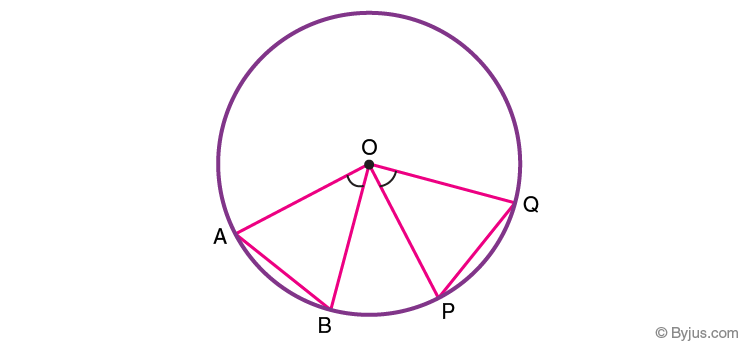Proof:

From fig. 4, In ∆AOB and ∆POQ

S.No. Statement Reason
1. ∠AOB = ∠POQ Equal angle subtended at centre O (Given)
2. OA = OB = OP = OQ Radii of the same circle
3. △AOB ≅ △POQ SAS axiom of Congruence
4. AB = PQ From Statement 3 (CPCT)

### Theorem 3: Equal Chords Equidistant from Center Theorem

Statement: Equal chords of a circle are equidistant from the center of the circle.

Proof:

Given: Chords AB and CD are equal in length.

Construction: Join A and C with centre O and drop perpendiculars from O to the chords AB and CD.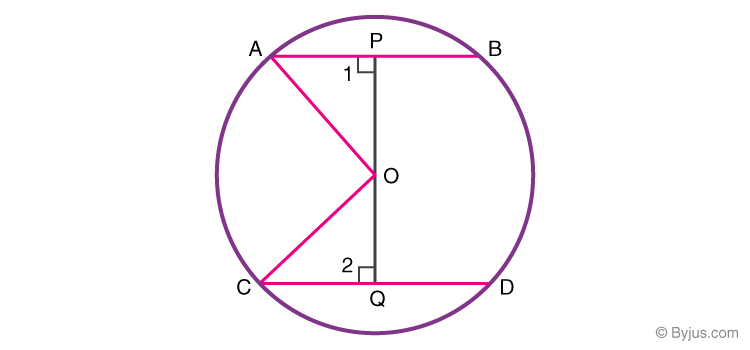S.No. Statement Reason
1 AP = AB/2, CQ = CD/2 The perpendicular from centre bisects the chord
In △OAP and △OCQ
2 ∠1 = ∠2 = 90° OP⊥AB and OQ⊥CD
3 OA = OC Radii of the same circle
4 OP = OQ Given
5 △OPB ≅ △OQD R.H.S. Axiom of Congruency
6 AP = CQ Corresponding parts of congruent triangle
7 AB = CD From statement (1) and (6)

### What is a Circle?

A circle is defined as a closed two-dimensional figure whose all the points in the boundary are equidistant from a single point (called centre).

### What is the Chord of a Circle?

The chord is a line segment that joins two points on the circumference of the circle. A chord only covers the part inside the circle.

### What is the Formula of Chord Length?

The length of any chord can be calculated using the following formula:

Chord Length = 2 × √(r2 − d2)

### Is Diameter a Chord of a Circle?

Yes, the diameter is also considered as a chord of the circle. The diameter is the longest chord possible in a circle and it divides the circle into two equal parts.

Quiz on Chord of a Circle

This link is excellent effort to learn maths

2. IF I know the length of the arc and the height of the arc. What formula can I use to calculate chord length?

1. lavanya

Let r is the radius, a is the arc length and h is the height of the arc.
h = r±√(r^2-l^2)
where is l is half of the length of the chord.
l = r sin(a/2r)

3. Caleb obakayode

Is there the area of a chord???

1. lavanya

A chord is a straight line whose endpoints lie on the circle. Therefore, a line cannot have an area.